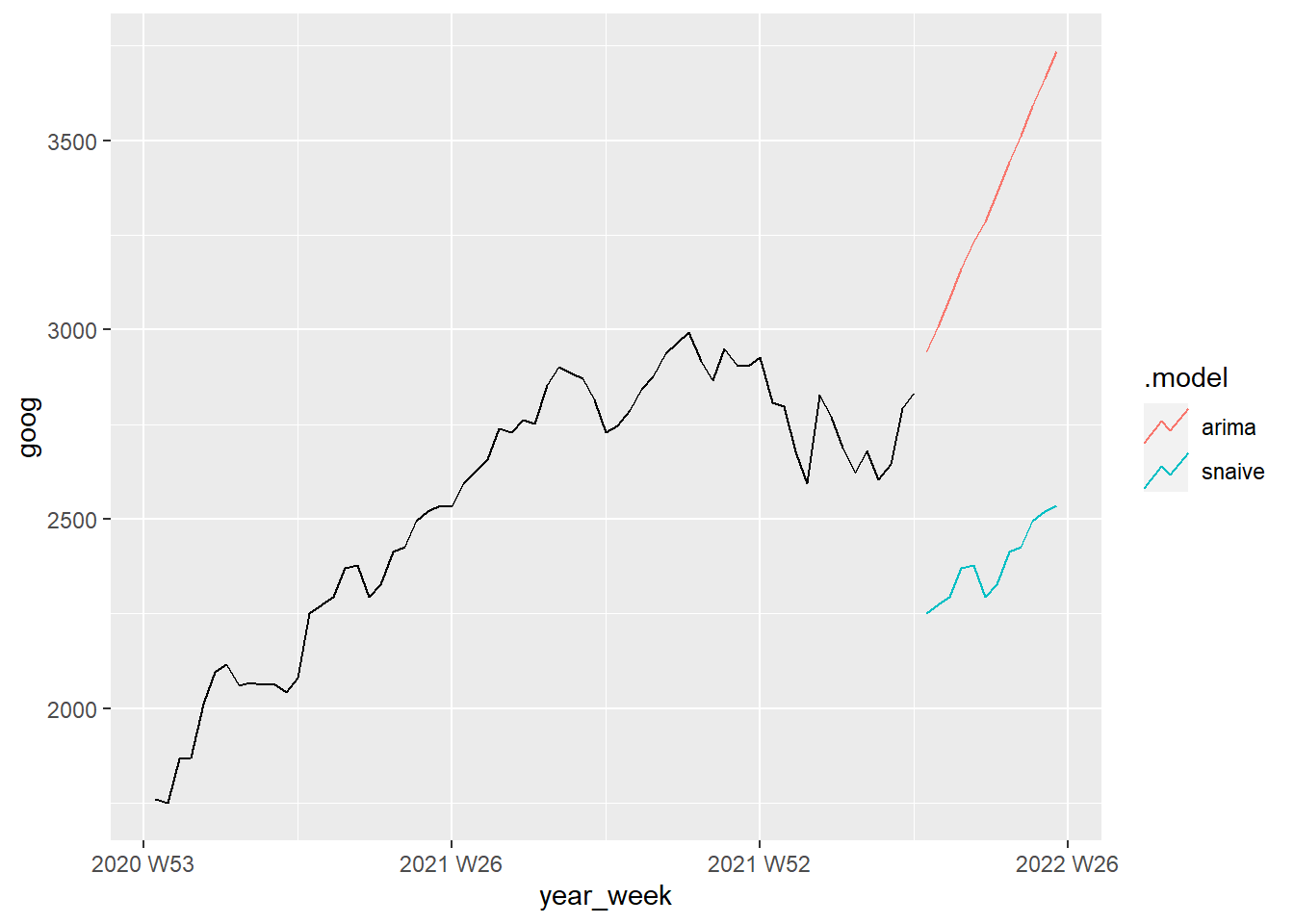The purpose of this in-class lab is to use R to practice with time series forecasting. The lab should be completed in your group. To get credit, upload your .R script to the appropriate place on Canvas.

## For starters

Open up a new R script (named ICL14_XYZ.R, where XYZ are your initials) and add the usual “preamble” to the top:

# Add names of group members HERE
library(tidyverse)
library(wooldridge)
library(broom)
library(magrittr)
library(modelsummary)
library(tsibble)
library(pdfetch)
library(tseries)
library(lubridate) # This package converts dates to a special date numbering system
library(fable)     # This one may take awhile to install
library(feasts)    # You also need to install this one
library(urca)      # and this one

First we’ll look at the return on a 3-month treasury bill over the period of 1960q1–1990q4. Second, we’ll read in Google’s and Apple’s stock price data from January 3, 2005 until April 4, 2022.

# T-bill rates by quarter
df1 <- as_tibble(intqrt)
df1 %<>% mutate(quarter = seq(yq('1960:Q1'),yq('1990:Q4'), by = 'quarters')) # create quarter
df1 %<>% select(r3,quarter)

# Stock prices
df2 <- pdfetch_YAHOO(c("goog","aapl"), fields = c("adjclose"),
from = as.Date("2005-01-01"),
to = as.Date("2022-04-04"),
interval = "1d") %>%
as.data.frame %>% rownames_to_column(var="date") %>%
as_tibble %>% mutate(date=ymd(date)) # create date variable

### Declare as time series objects

df1 %<>% as_tsibble(index=quarter)
df2 %<>% as_tsibble(index=date) %>% # aggregate from daily to weekly
index_by(year_week = yearweek(date)) %>%
summarize(goog = mean(goog, na.rm=TRUE),
aapl = mean(aapl, na.rm=TRUE))

## Plot time series data

Let’s have a look at the 3-month T-bill return for the US over the period 1960–1990:

autoplot(df1) + xlab("Year") + ylab("T-bill return")
## Plot variable not specified, automatically selected .vars = r3

autoplot(df2) + xlab("Year") + ylab("Price")
## Plot variable not specified, automatically selected .vars = goog

## Testing for a unit root

Let’s test for a unit root in each of the time series. The way to do this is the Augmented Dickey-Fuller (ADF) test, which is available as adf.test() in the tseries package.

The function tests $$H_0: \text{Unit Root}, H_a: \text{Stationary}$$.

adf.test(df1$r3, k=1) adf.test(df2$goog, k=1)
## Warning in adf.test(df2$goog, k = 1): p-value greater than printed p-value adf.test(df2$aapl, k=1)
## Warning in adf.test(df2$aapl, k = 1): p-value greater than printed p-value 1. Which of these time series has a unit root, according to the ADF test? Explain what the consequences are of analyzing a time series that contains a unit root. ### Estimating AR(1) models To alternatively examine the unit root, we can estimate AR(1) models for each series: est.tbill <- lm(r3 ~ lag(r3,1), data=df1) est.goog <- lm(goog ~ lag(goog,1), data=df2) est.aapl <- lm(aapl ~ lag(aapl,1), data=df2) modelsummary(list(est.tbill,est.goog,est.aapl)) 1. Are the $$R^2$$ values from these estimates meaningful? ## Forecasting Now let’s use our time series data to forecast future stock prices. First, we should create a shortened version of the time series so we can compare our forecast to actual data: df2.short <- df2 %>% filter(year_week<yearweek("2022-01-01")) ### Estimating simple ARIMA(1,1,0) models simple.goog <- lm(difference(goog) ~ lag(difference(goog)), data=df2) simple.aapl <- lm(difference(aapl) ~ lag(difference(aapl)), data=df2) which is estimating $\Delta goog_t = \rho \Delta goog_{t-1} + u_t$ ### Estimating ARIMA models We can also use the ARIMA function in the fable package to allow the computer to choose the best ARIMA model: auto.goog <- ARIMA(df2.short$goog)
auto.aapl <- ARIMA(df2.short\$aapl)

### Plotting forecasts

We can compare the 90-day-ahead (12-week-ahead) forecasts of each model by looking at their plots:

df2 %>%
model(
arima = ARIMA(goog),
snaive = SNAIVE(goog)
) %>%
forecast(h=12) %>% autoplot(filter(df2, year(year_week)>2020),level = NULL)